# 【语音去噪】基于改进谱减法语音去噪matlab 源码

• 时间:
• 浏览:

## 一、简介

（1）对谱减算法中的负数部分进行了非线性处理
（2）对噪声谱的估计不准
（3）抑制函数（增益函数）具有较大的可变性
1 原理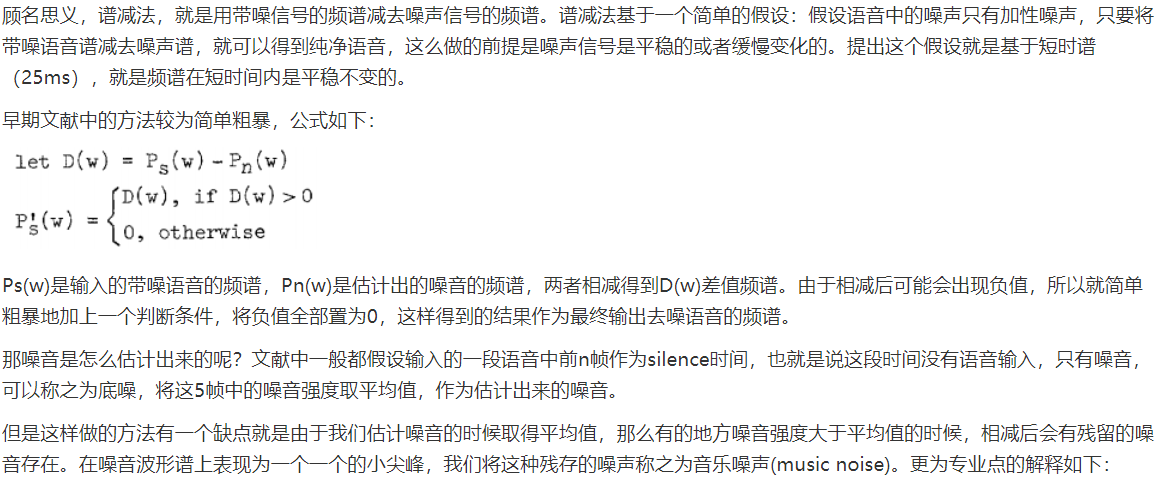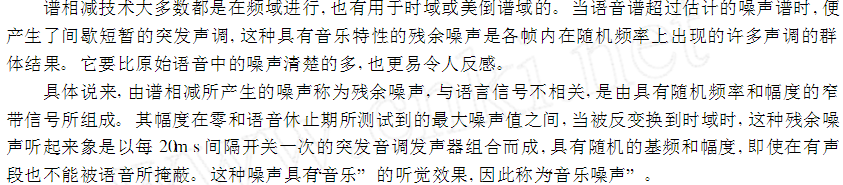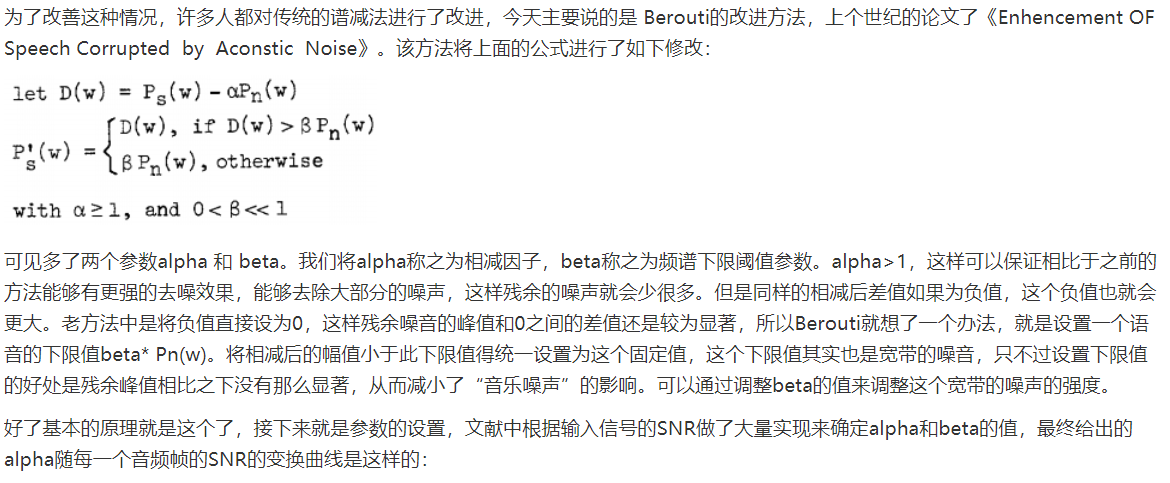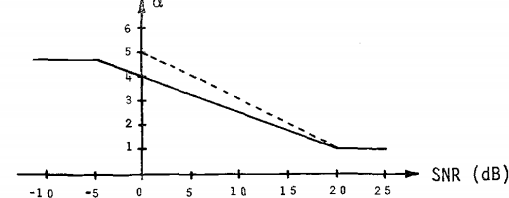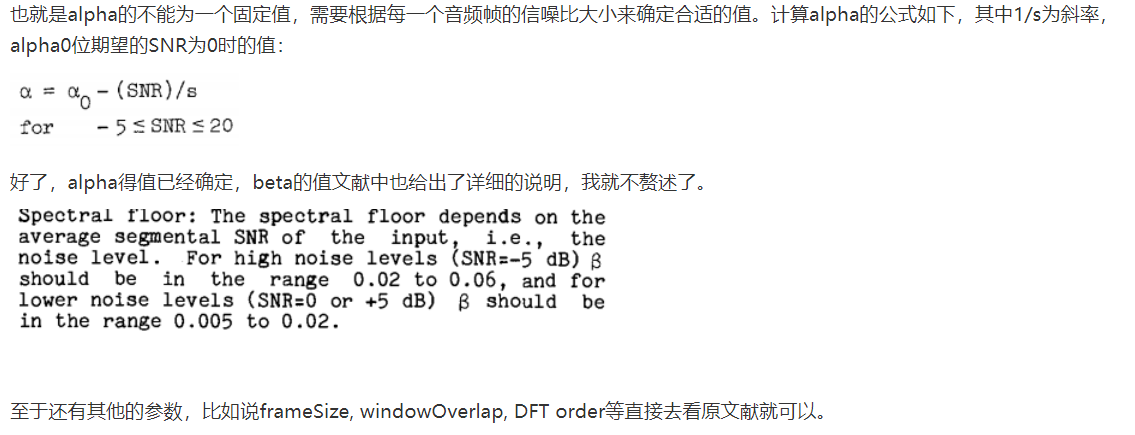## 二、源代码

``````clear all; clc; close all;

xx=xx-mean(xx);                         % 消除直流分量
x=xx/max(abs(xx));                      % 幅值归一化
SNR=10;                                 % 设置信噪比
signal=awgn(x,SNR,'measured','db');                % 叠加噪声
snr1=SNR_Calc(x,signal);            % 计算叠加噪声后的信噪比
N=length(x);                            % 信号长度
time=(0:N-1)/fs;                        % 设置时间刻度
IS=.15;                                 % % 设置前导无话段长度
wlen=200;                               % 设置帧长为25ms
inc=80;                                 % 设置帧移为10ms
Gamma=1;                                %幅度加权（改进谱减法的参数）
Beta=.03;
NIS=fix((IS*fs-wlen)/inc +1);           % 求前导无话段帧数
output=SpectralSubIm(signal,wlen,inc,NIS,Gamma,Beta);          % 调用SSBoll79函数做谱减
output=output/max(abs(output));
ol=length(output);                      % 把output补到与x等长
if ol<N
output=[output; zeros(N-ol,1)];
end
function frameout=enframe(x,win,inc)

nx=length(x(:));            % 取数据长度
nwin=length(win);           % 取窗长
if (nwin == 1)              % 判断窗长是否为1，若为1，即表示没有设窗函数
len = win;               % 是，帧长=win
else
len = nwin;              % 否，帧长=窗长
end
if (nargin < 3)             % 如果只有两个参数，设帧inc=帧长
inc = len;
end
function snr=SNR_Calc(I,In)
% 计算带噪语音信号的信噪比
% I 是纯语音信号
% In 是带噪的语音信号
% 信噪比计算公式是
% snr=10*log10(Esignal/Enoise)
I=I(:)';                             % 把数据转为一列
In=In(:)';
function frameout=filpframe(x,win,inc)

[nf,len]=size(x);
nx=(nf-1) *inc+len;                 %原信号长度
frameout=zeros(nx,1);
nwin=length(win);                   % 取窗长
if (nwin ~= 1)                           % 判断窗长是否为1，若为1，即表示没有设窗函数
winx=repmat(win',nf,1);
x=x./winx;                          % 除去加窗的影响
x(find(isinf(x)))=0;                %去除除0得到的Inf
end
``````

## 三、运行结果## 四、备注

### 往期回顾>>>>>>

【信号处理】基于LMS算法信号去噪matlab源码

【语音去噪】基于先验信噪比的维纳滤波算法语音去噪matlab源码

【信号去噪】基于谱减法去噪matlab源码

【语音去噪】基于基本维纳滤波算法语音去噪matlab源码